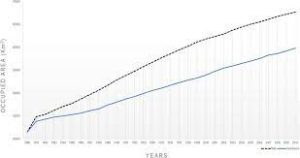## How to Convert Square Yards to Square Kilometers | Area Units

The image below represents square yards to square kilometers.To convert square yards to square kilometers, one essential parameter is needed and this parameter is Unit Value.

The formula for converting square yards to square kilometers:

1 Square Yard = 0.00000083612736 Square Kilometers

Let’s solve an example;
Find the conversion of square yards to square kilometers when the unit value is 14.

1 Square Yard = 0.00000083612736 Square Kilometers

Then,

14 Square Yards = (14 x 0.00000083612736) Square Kilometers
14 Square Yards = 0.00001170578304 Square Kilometers

Therefore, the square kilometer(s) is 0.0000117.